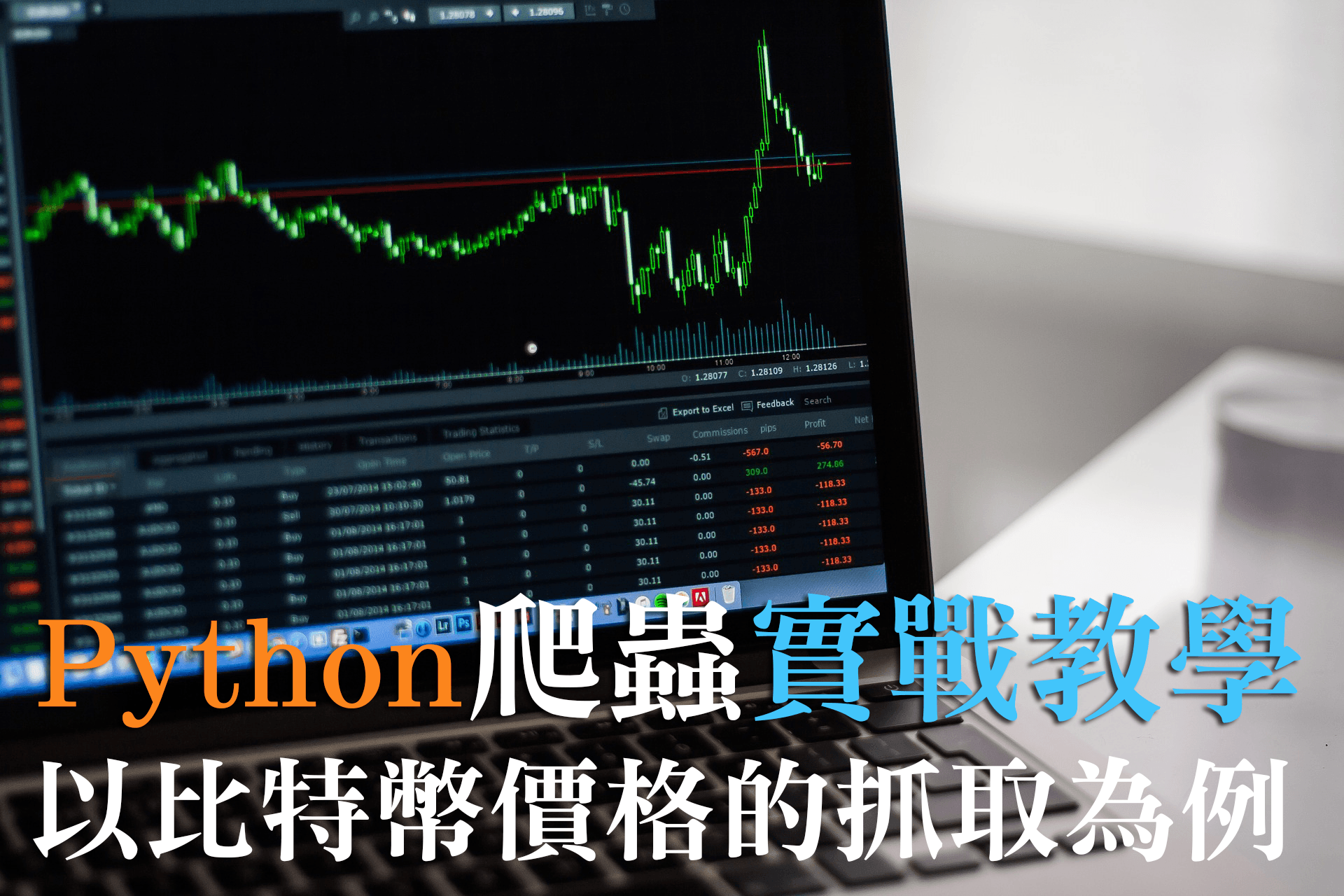## Step0 待會需要用到的模組：

1. pandas
2. requests
3. matplotlib

```pip install pandas
pip install requests
pip install matplotlib```

## Step1 鎖定用以抓取的網站：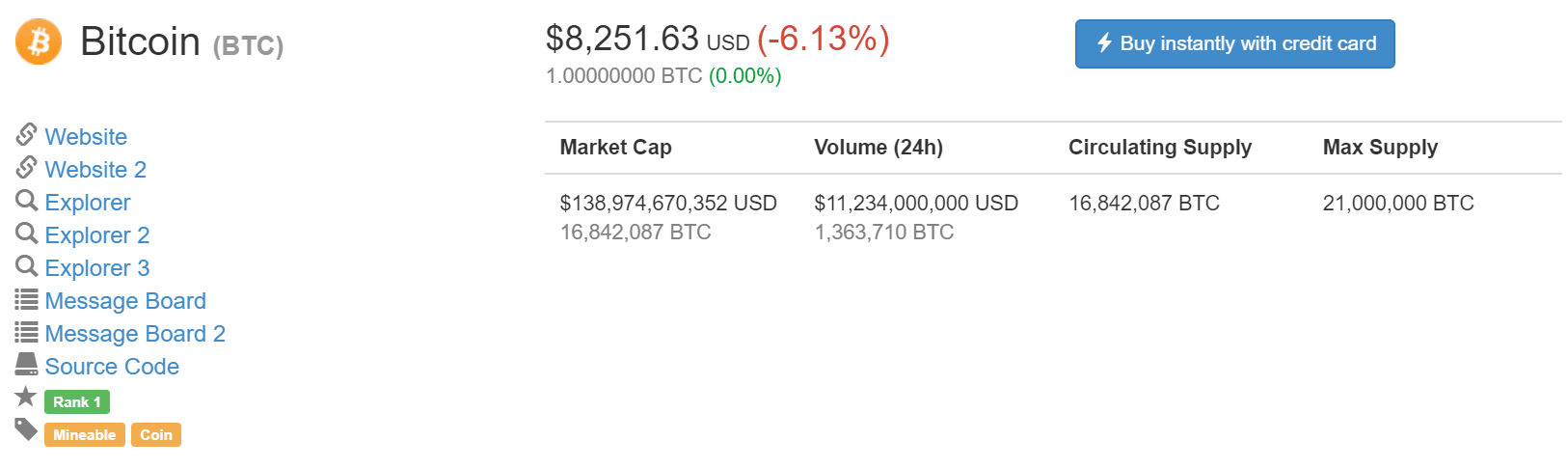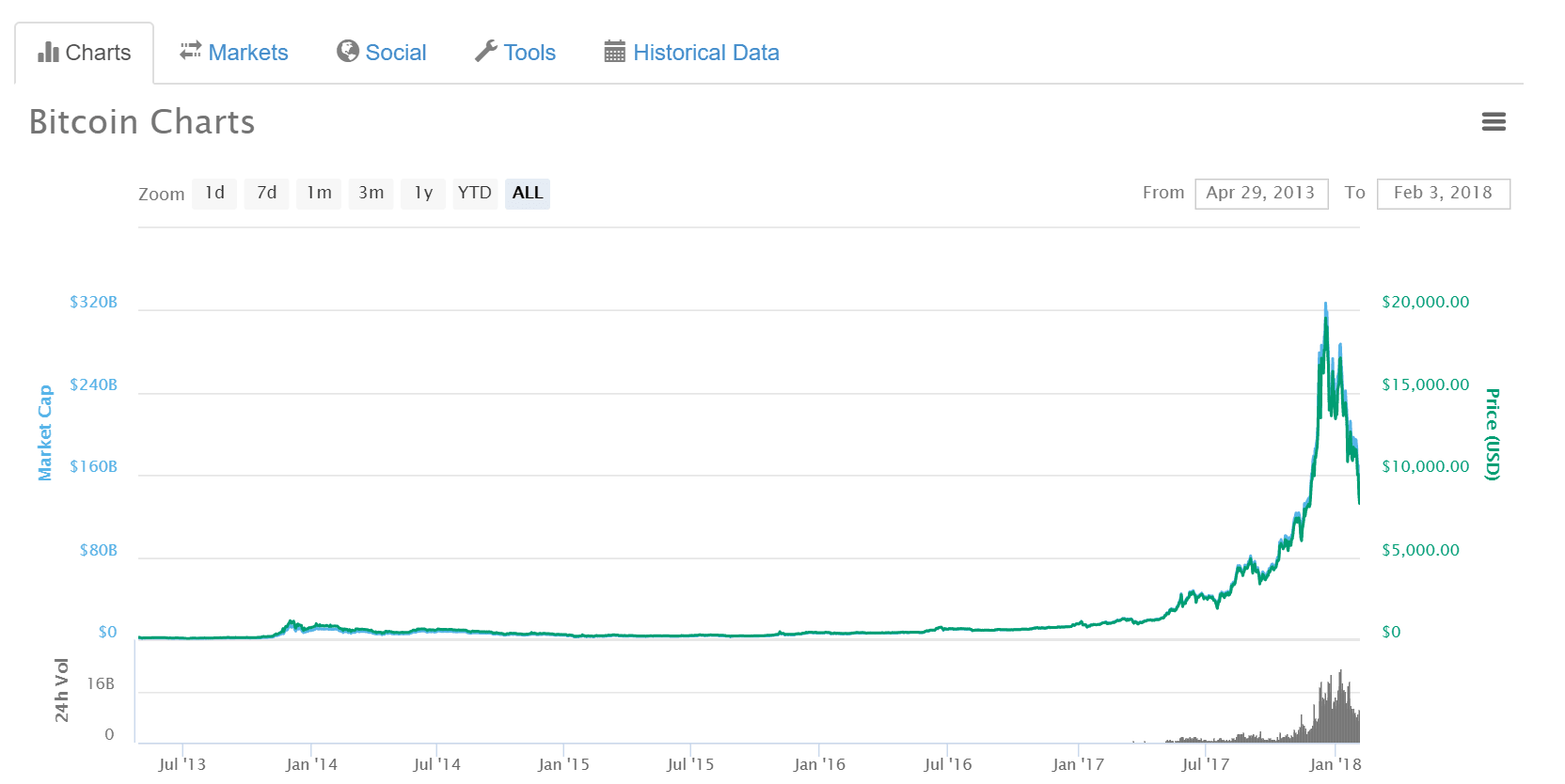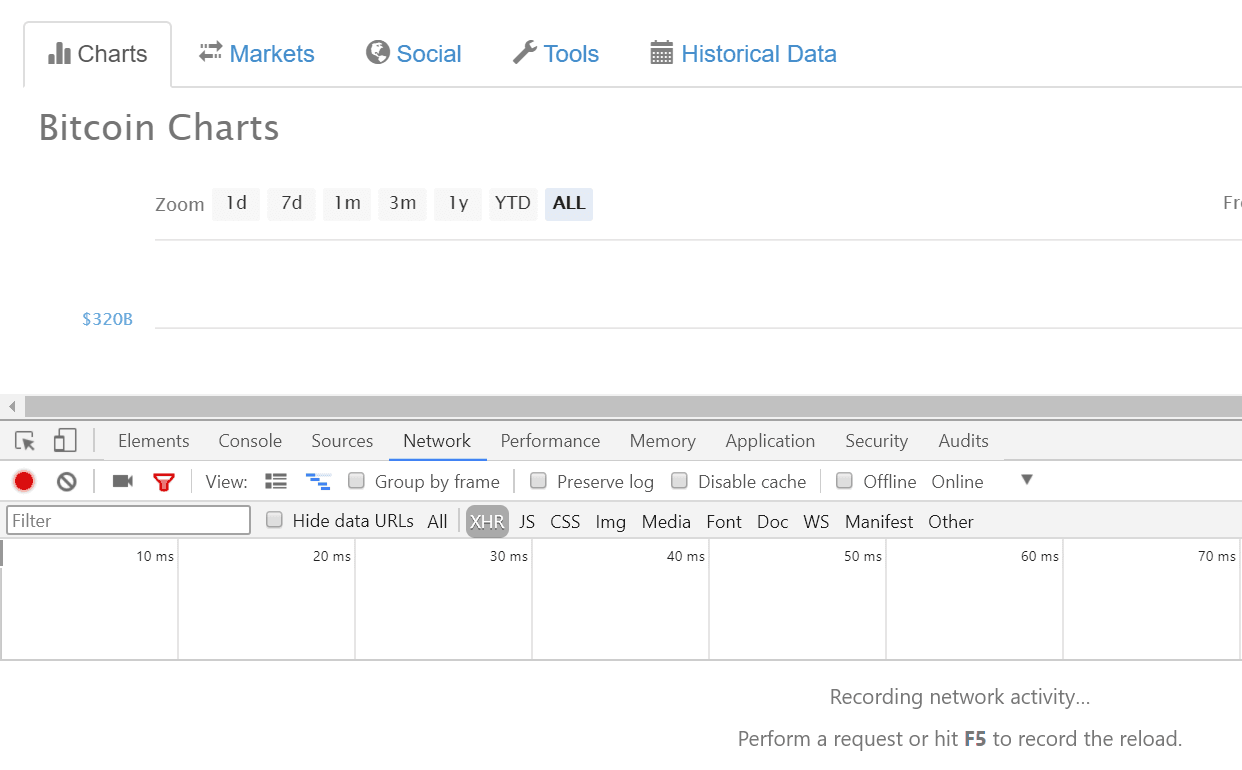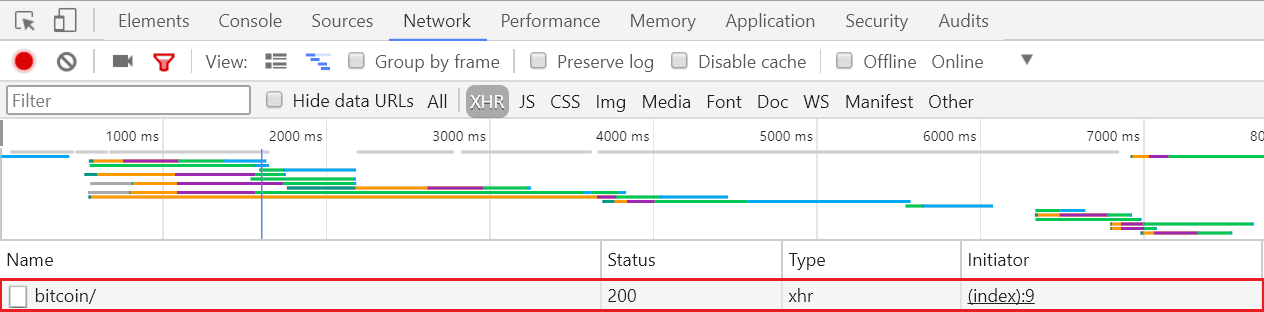Response 頁籤中，可以看到 market_cap_by_available_supply ，但裡頭未必包含我們想要的資訊，因此可以再點左邊的 Preview 來看看裡面大致包含了什麼東西。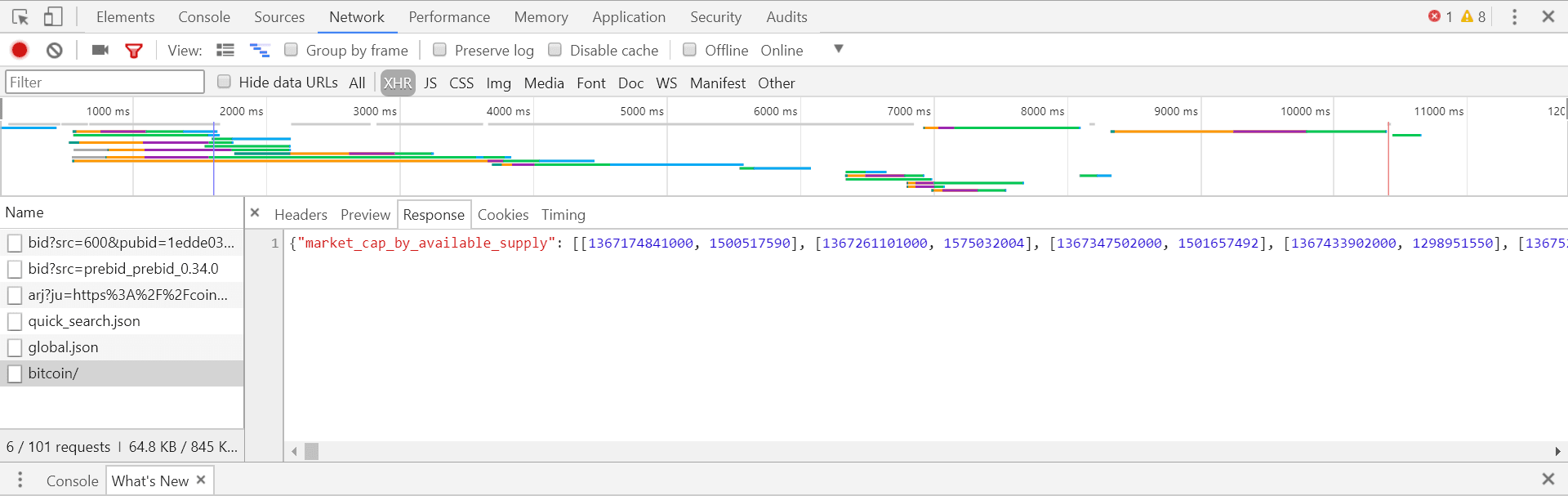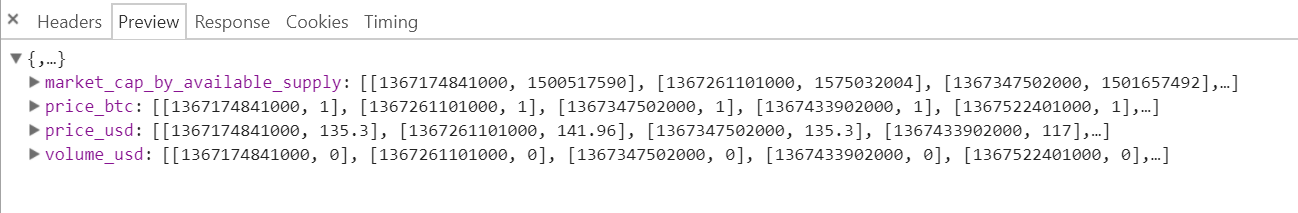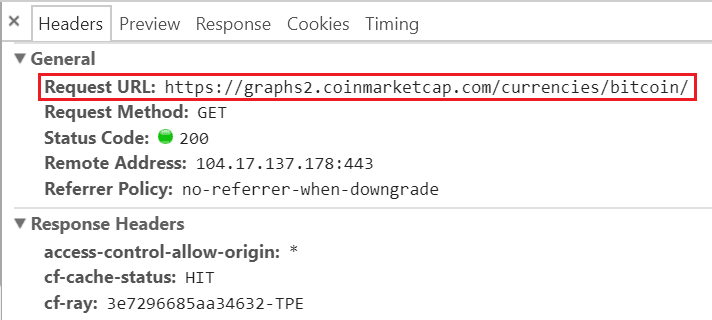## Step2 以Python爬取資訊：

```import requests
import pandas
import matplotlib.pyplot as plt```

`res = requests.get('https://graphs2.coinmarketcap.com/currencies/bitcoin/') #將取得的結果放入res中`

requests模組甚至提供了json()函數，可將取得的結果直接轉為python的內建資料型態 dictionary。

`d = res.json()`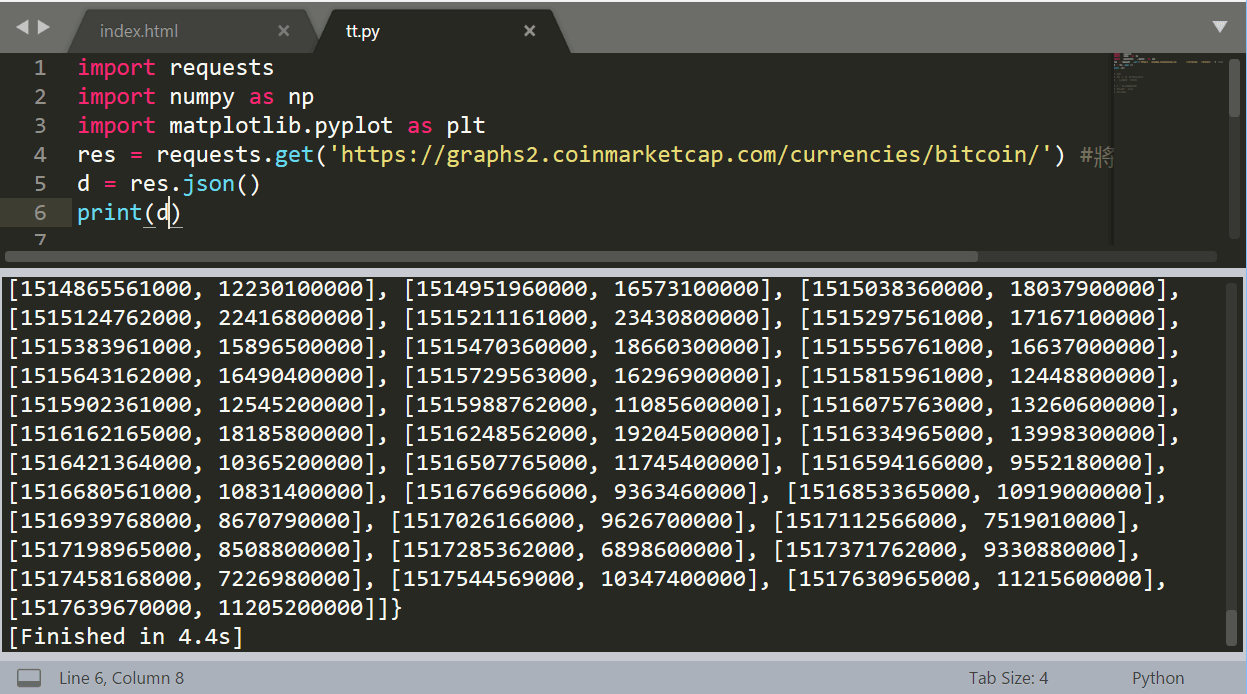`df = pandas.DataFrame(d)`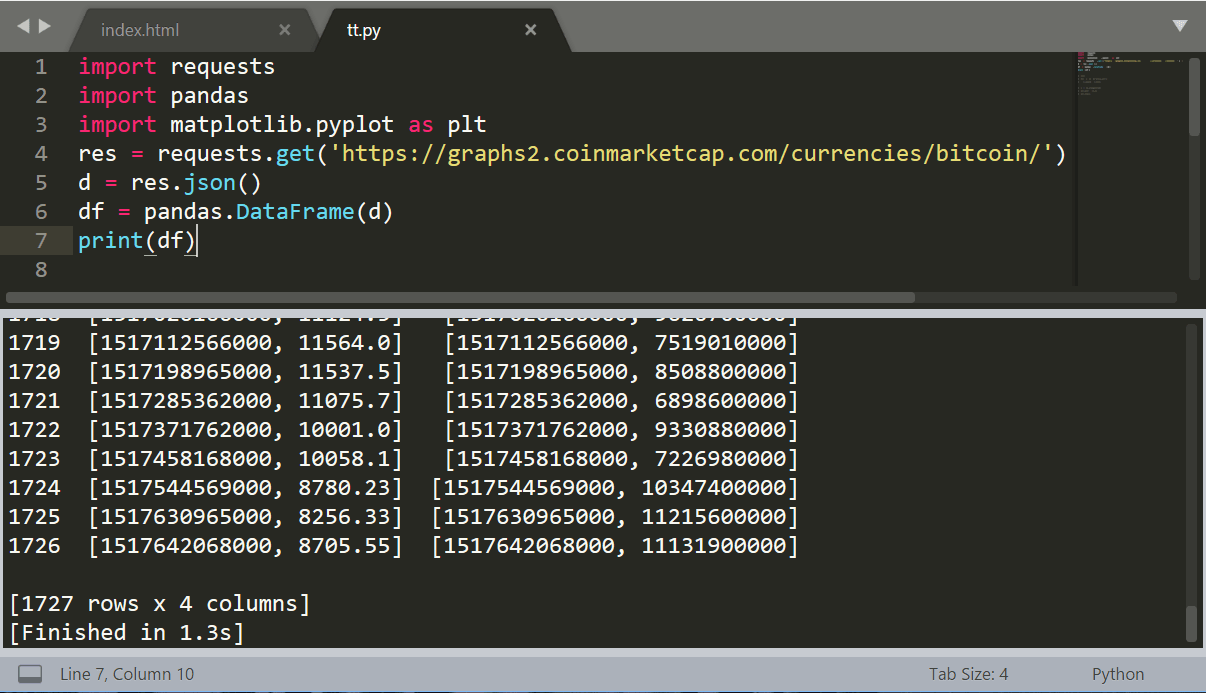`print(df['price_usd'])`

```df = df['price_usd']
date=[]
price=[]
for i in df:
i = pandas.to_datetime(i,unit='ms')
date.append(i)
price.append(i)```

## Step3 以 Matplotlib 繪製價錢走勢圖：

```plt.plot(date,price)
plt.show()```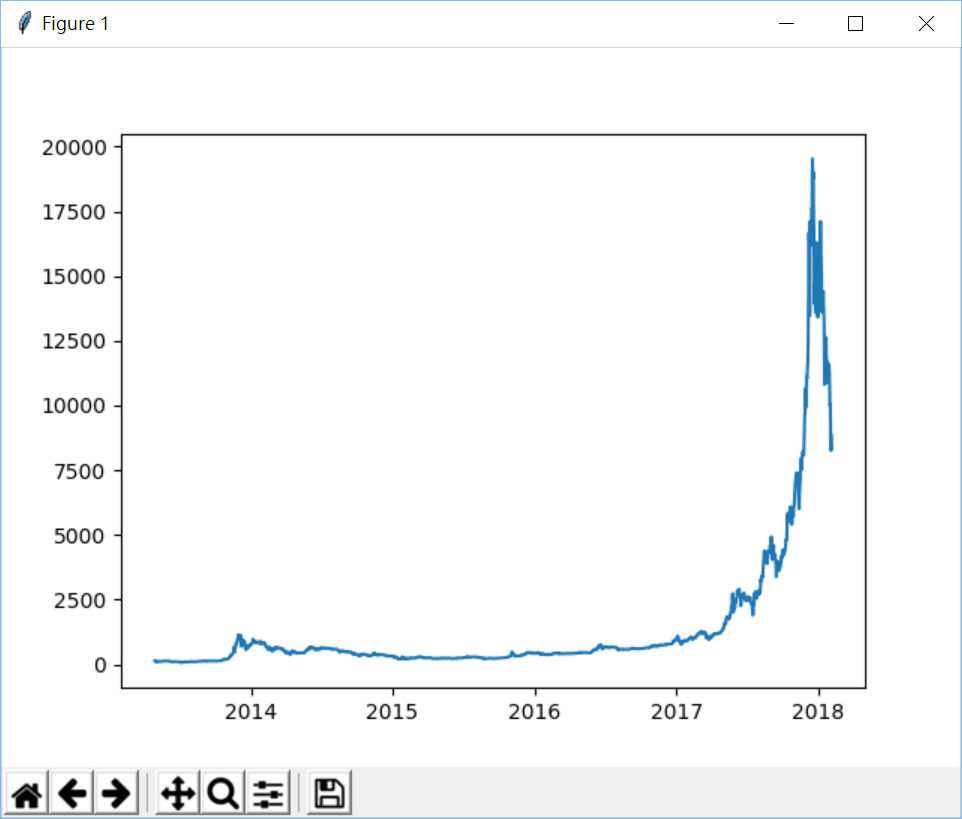```plt.plot(date,price)
plt.xlabel('Date')
plt.ylabel('Price (USD)')
plt.title('Price of Bitcoin')
plt.show()```

• 12
•
•
•
•
12
Shares